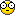# Write a program to determine if a binary tree is well ordered##Write a program to determine if a binary tree is well ordered

public static boolean judge(TreeNode root){
if(root==null)
return false;
if(root.left){
if(root.left.value>=root.value)
return false;
judge(root.left);
}
if(root.right){
if(root.right.value<=root.value)
return false;
judge(root.right);
}
return true;
}

Code from CareerCup:

boolean verifyBST(Node* aNode)
{
if((aNode->left) && (aNode->left->value > aNode->value))
return false;
if((aNode->right) && (aNode->right->value < aNode->value))
return false;

if(!(aNode->left&&aNode->right)) return true;
else {
if(verifyBST(aNode->left) && verifyBST(aNode->right))
return true;
else
return false;
}
}

##Re: Write a program to determine if a binary tree is well ordered

I assume by CareerCup, you mean the website, not the book, right?

Anyway, both solutions are very wrong. Actually, in my third round Amazon phone interview, I made the same mistake and was rejected.Please think for a moment why it is wrong.

##Re: Write a program to determine if a binary tree is well ordered

I have checked the second code and did not consider the aNode is NULL case
Here is the revised version:

boolean verifyBST(Node* aNode){
if(aNode==null)
return true;
if((aNode->left) && (aNode->left->value > aNode->value))
return false;
if((aNode->right) && (aNode->right->value < aNode->value))
return false;

if(!(aNode->left&&aNode->right)) return true;
else {
if(verifyBST(aNode->left) && verifyBST(aNode->right))
return true;
else
return false;
}
}

##Re: Write a program to determine if a binary tree is well ordered

Revise for the first code:

public static boolean judge(TreeNode root){
if(root==null)
return true;
if(root.left){
if(root.left.value>=root.value)
return false;
}

if(root.right){
if(root.right.value<=root.value)
return false;
}

if(judge(root.left)&&judge(root.right))
return true;
else
return false;
}

Check me if I'm wrong##Re: Write a program to determine if a binary tree is well ordered

No, the error is not some corner cases. There are more fundamental problems there. Think about the definition of BST.

##Re: Write a program to determine if a binary tree is well ordered

Is that all the node in left tree are smaller than the root node, and all the node in right tree are bigger than the root node?

viterbi wrote:No, the error is not some corner cases. There are more fundamental problems there. Think about the definition of BST.

##Re: Write a program to determine if a binary tree is well ordered

OK. I will give more hint. Think: is this a BST?

15
|\
10 18
|\
8 17

What will the answer of your program?

Admin wrote:Is that all the node in left tree are smaller than the root node, and all the node in right tree are bigger than the root node?

viterbi wrote:No, the error is not some corner cases. There are more fundamental problems there. Think about the definition of BST.

##Re: Write a program to determine if a binary tree is well ordered

Yes, that is what I mean, the left tree has node greater than the root, so it is not BST

viterbi wrote:OK. I will give more hint. Think: is this a BST?

15
|\
10 18
|\
8 17

What will the answer of your program?

Admin wrote:Is that all the node in left tree are smaller than the root node, and all the node in right tree are bigger than the root node?

viterbi wrote:No, the error is not some corner cases. There are more fundamental problems there. Think about the definition of BST.

##Re: Write a program to determine if a binary tree is well ordered

But your program will return true.

Admin wrote:Yes, that is what I mean, the left tree has node greater than the root, so it is not BST

viterbi wrote:OK. I will give more hint. Think: is this a BST?

15
|\
10 18
|\
8 17

What will the answer of your program?

Admin wrote:Is that all the node in left tree are smaller than the root node, and all the node in right tree are bigger than the root node?

viterbi wrote:No, the error is not some corner cases. There are more fundamental problems there. Think about the definition of BST.

##Re: Write a program to determine if a binary tree is well ordered

We can implement a in-order-traversal to list all elements in an array and to judge whether the array is in ascending order.

Time complexity O(n)
Space complexity O(n)

Check me if I'm wrong

viterbi wrote:But your program will return true.

Admin wrote:Yes, that is what I mean, the left tree has node greater than the root, so it is not BST

viterbi wrote:OK. I will give more hint. Think: is this a BST?

15
|\
10 18
|\
8 17

What will the answer of your program?

Admin wrote:Is that all the node in left tree are smaller than the root node, and all the node in right tree are bigger than the root node?

viterbi wrote:No, the error is not some corner cases. There are more fundamental problems there. Think about the definition of BST.

##Re: Write a program to determine if a binary tree is well ordered

This is correct, but it need an additional array, right?

Admin wrote:We can implement a in-order-traversal to list all elements in an array and to judge whether the array is in ascending order.

Time complexity O(n)
Space complexity O(n)

Check me if I'm wrong

viterbi wrote:But your program will return true.

Admin wrote:Yes, that is what I mean, the left tree has node greater than the root, so it is not BST

viterbi wrote:OK. I will give more hint. Think: is this a BST?

15
|\
10 18
|\
8 17

What will the answer of your program?

Admin wrote:Is that all the node in left tree are smaller than the root node, and all the node in right tree are bigger than the root node?

viterbi wrote:No, the error is not some corner cases. There are more fundamental problems there. Think about the definition of BST.

##Re: Write a program to determine if a binary tree is well ordered